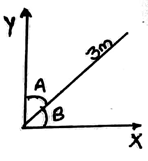Q.1
##### Which of the following statement is true?
• a) A scalar is any physical quantity that can be completely specified by its magnitude
• b) A vector is any positive or negative physical quantity that can be completely specified by its magnitude
• c) A scalar is any physical quantity that requires both a magnitude and a direction for its complete description
• d) A scalar is any physical quantity that can be completely specified by its direction
Q.2
##### For two vectors defined by an arrow with a head and a tail. The length of each vector and the angle between them represents:
• a) Their magnitude’s square and direction of the line of action respectively
• b) Their magnitude and direction of the line of action respectively
• c) Magnitude’s square root and direction of the line of action respectively
• d) Magnitude’s square and the ratio of their lengths respectively
Q.3
##### If a vector is multiplied by a scalar:
• a) Then its magnitude is increased by the square root of that scalar’s magnitude
• b) Then its magnitude is increased by the square of that scalar’s magnitude
• c) Then its magnitude is increased by the amount of that scalar’s magnitude
• d) You cannot multiply the vector with a scalar
Q.4
##### All the vectors quantities obey:
• a) Parallelogram law of addition
• b) Parallelogram law of multiplication
• c) Parallelogram law of addition of square root of their magnitudes
• d) Parallelogram law of addition of square of their magnitudes
Q.5
##### A force vector with magnitude R and making an angle α with the x-axis is having its component along x-axis and y-axis as:
• a) Rcosine (α) and Rsine(α)
• b) Rcosine (180-α) and Rsine(α)
• c) Rcosine (180-α) and Rsine(180+α)
• d) Rcosine (α) and Rsine(180+α)
Q.6
• a) Cot α
• b) Tan α
• c) Sec α
• d) 1
Q.7
##### Vector shown in the figure below have a length ofand the angles shown A and B areanddegrees each. Calculate the X-axis and Y-axis components:• a) 2.59m and 1.50m respectively
• b) 1.50m and 2.59m respectively
• c) 3cos60 and 3sin30 respectively
• d) 3sin60 and 3sin30 respectively
Q.8
• a) 3.23m
• b) 4.35m
• c) 2.50m
• d) 1.5m
Q.9
##### The magnitude of the resultant of the two vectors is always_____________
• a) Greater than one of the vector’s magnitude
• b) Smaller than one of the vector’s magnitude
• c) Depends on the angle between them
• d) Axis we choose to calculate the magnitude
Q.10
##### If two equal vector forces are mutually perpendicular then the resultant force is acting at which angle as compared to one of the vector?
• a) 45 degree
• b) 90 degree
• c) 180 degree
• d) 0 degree
Q.11
##### What is the direction of the resultant vector if two vectors having equal length is placed in the Cartesian plane at the origin as, one being parallel to and heading towards positive x-axis and the other makingdegree with it and heading in the opposite direction of that of the first one?
• a) It is either in the 1st quadrant or in the 2nd quadrant
• b) It is either in the 1st quadrant or in the 3rd quadrant
• c) It is either in the 1st quadrant or in the 4th quadrant
• d) Only in the 1st quadrant
Q.12
##### Force vector R is having a______________
• a) Length of R and a specific direction
• b) Length of R
• c) A specific direction
• d) Length of magnitude equal to square root of R and a specific direction
Q.13
• a) True
• b) False
Q.14
• a) True
• b) False